´

## Group selection - Choose prescribed automorphism group

If you choose the "group" button in DISCRETA you have different possibilities for further choices. You get the following list: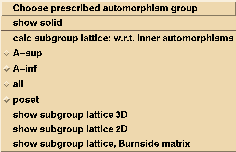Here you have to press "Choose prescribed automorphism group" to receive a list of groups we have implemented. A new window will open: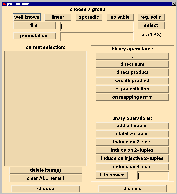Besides the possibility of typing in a set of generating permutations by hand (an example is given) you have the choice between 5 kinds of groups:

 The first selection is "well known". Here you can choose the symmetric, the alternating, dieder or the cyclic group on n points (you have to choose the parameter n first), as well as the holomorph of the cyclic group on n points, the trivial group, the (only) subgroup of Hol(Cn) of index m or finally the wreath product of Sn and Sm.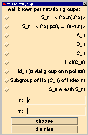For the construction of designs very useful are the linear groups - you have to press "linear": we consider the following matrix groups in their permutation representation. First of all there is the general linear group GL(n,q) - where q is the order of the corresponding field - of regular n /times n-matrices; then SL(n,q) is the special linear group of matrices with determinante 1. Derived from them we have the GGL(n,q) where GG stands for Gamma, and the SSl(n,q) where SS stands for Sigma. The corresponding projective groups we get as factor groups modulo the center. Then we have the affine groups of affine transformations. Finally, you can choose the group of affine translations T(n,q), the projective special unitary group PSU(3,q2) and the Sz(q). For all groups you have to choose the parameters n and q.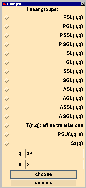You also can use several sporadic groups: The Mathieu groups M11, M12, M23 and M24 are simple groups. They are the only nontrivial, at least 4-transitive groups: M11: sharply 4-transitive of degree 11, order: 11 · 10 · 9 · 8 M12: sharply 5-transitive of degree 12, order: 12 · 11 · 10 · 9 · 8 M23: 4-transitive of degree 23, order: 23 · 22 · 21 · 20 · 3 · 24 M24: 5-transitive of degree 24, order: 24 · 23 · 22 · 21 · 20 · 3 · 24 The Higman-Sims group HS can be described as the automorphism group of a combinatorial geometry consisting of a set M of 176 points and a set Q of 176 quadrics. It is 2-transitive in its action on M and has an equivalent 20transitive action on Q. The order of HS is 44.352.000.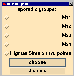There exists a catalogue of all solvable groups of degree at most 127. With the parameter n you choose the degree and the choice of m gives you the m-th group of degree n in the catalogue.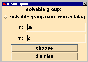Here you can choose the symmetry groups of regular and semiregular solids. You start with the choice of one of the platonic solids: tetrahedron, cube, octahedron, icosahedron and dodecahedron. Then it is possible to modify the chosen solid for example by truncating the edges (only for vizualizing the solid the distinction between dodecahedron, cube and the others is important - the group just the same, namely the induced group of the first solid), considering the dual solid i.e. taking the center of the faces as the new vertices (the platonic solids are pairwise dual: cube and octahedron, icosa- and dodecahedron, tetrahedron with itself); it is very interesting to build the dual of semiregular and archimedian solids, taking the midpoints of edges as the vertices of the new solid and adding a central point of a solid; that means adding a fixpoint of the automorphism group. In addition you can choose the group of the hypercube (4-dimensional cube). All those solids are drawn as 3D-graphs. The pictures can be shown on the screen by pressing the button "group" and "show solid" (examples).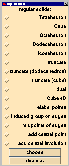Very useful are the combinations of the different groups. You have 2 possibilities:

• You can choose two groups and combine them by
• appending the second to the first
• building the direct sum
• building the direct product
• building the wreath product
• exponentiation of the first group by the second one
• considering the action on mappings nm
• You can choose one group and
• build a point stabilizer
• induce the action of the group on 2-sets
• induce the action of the group on 2-tuples
• induce the action of the group on the injective 2-tuples
• induce the action of the group on 3-sets
• build the i-th power of the group (you have to type in i)

One example (starting point is the tetrahedron) is given by the following selection: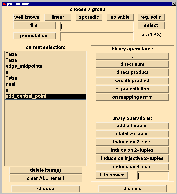The resulting designs and details of the construction you can find at this page.

Remark: You have to click on the pictures to get them largely.

Back to the description .
Back to the DISCRETA homepage .
Last updated: August 24, 1999, Evi Haberberger

University of Bayreuth -